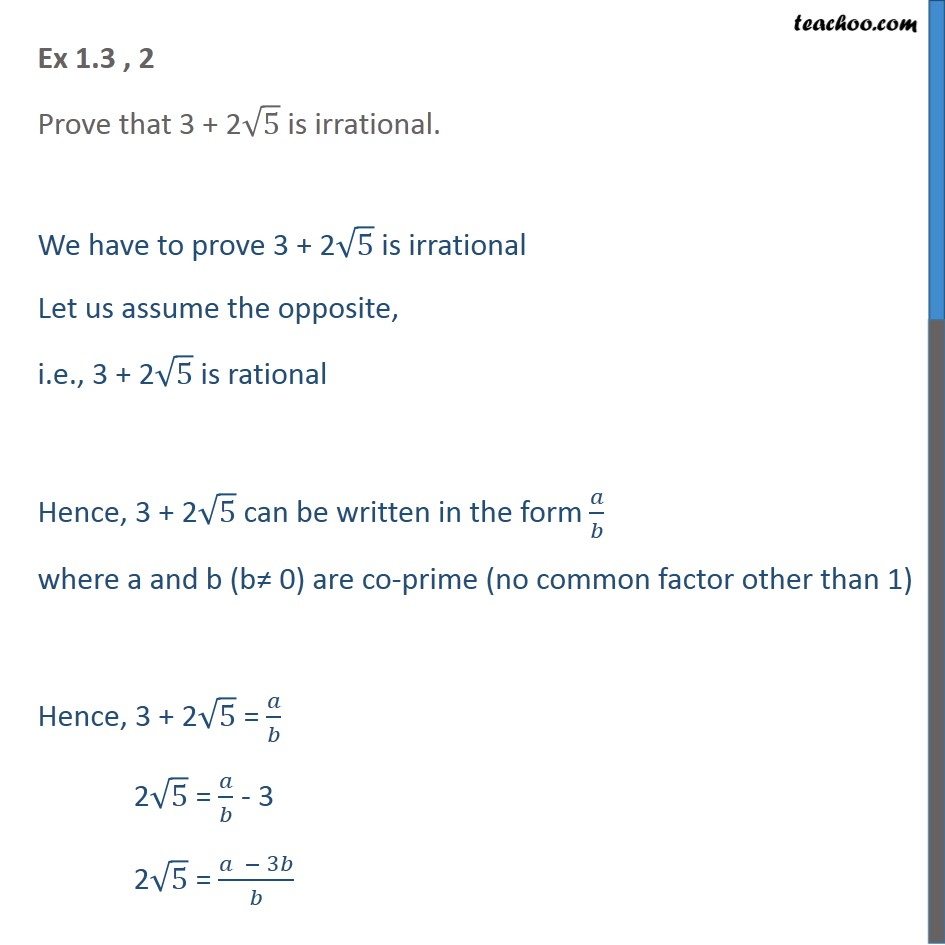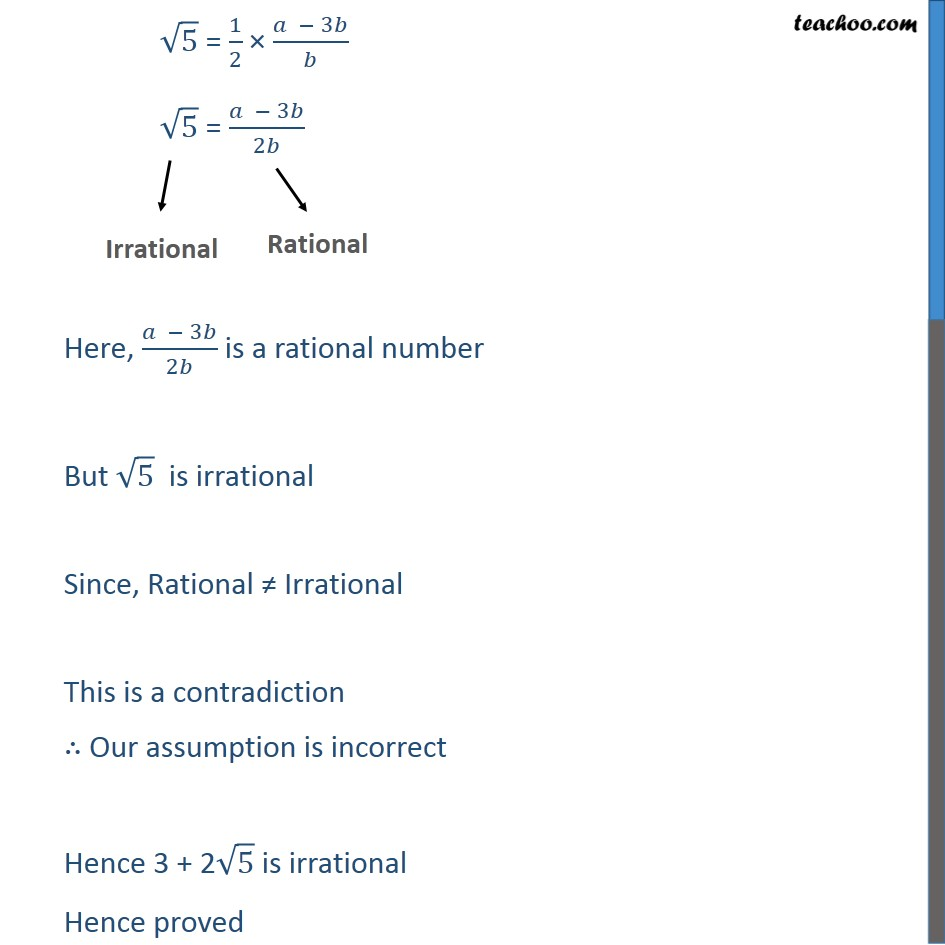Check Important Questions and Chapter Summary -https://you.tube/Real-Numbers-Class-101. Chapter 1 Class 10 Real Numbers
2. Concept wise
3. Irrational numbers

Transcript

Ex 1.3 , 2 Prove that 3 + 2 root 5 √5 is irrational. We have to prove 3 + 2 root 5√5 is irrational Let us assume the opposite, i.e., 3 + 2√5 is rational Hence, 3 + 2√5 can be written in the form 𝑎/𝑏 where a and b (b≠ 0) are co-prime (no common factor other than 1) Hence, 3 + 2√5 = 𝑎/𝑏 2√5 = 𝑎/𝑏 - 3 2√5 = (𝑎 − 3𝑏)/𝑏 √5 = 1/2 × (𝑎 − 3𝑏)/𝑏 √5 = (𝑎 − 3𝑏)/2𝑏 Here, (𝑎 − 3𝑏)/2𝑏 is a rational number But √5 is irrational Since, Rational ≠ Irrational This is a contradiction ∴ Our assumption is incorrect Hence 3 + 2√5 is irrational Hence proved

Irrational numbers